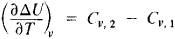# Kirchhoff's Equation

The following article is from The Great Soviet Encyclopedia (1979). It might be outdated or ideologically biased.

## Kirchhoff’s Equation

an equality expressing the temperature dependence of the thermal quantities associated with a chemical reaction through the difference in heat capacities between the products and reactants. Specifically, for reactions occurring at constant volume, Kirchhoff’s equation relates the temperature dependence of the change in internal energy ΔU of a reaction to the difference in isochoric heat capabilities Cν in the following form:where Cv,1 and Cv,2 are the sums of heat capacities of the reactants and products taking into account their stochiometric coefficients in the reaction equation. By integrating this equation, it is possible to determine the change in internal energy ΔUT at the temperature of interest T if the following are known: ΔUT1 at some other temperature T1 and the heat capacities of the reactants and products for the temperature range in question. Kirchhoff’s equation was deduced by G. R. Kirchhoff in 1858.

V. A. KIREEV